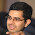## Tuesday, 23 October 2012

### Maximum and Minimum of array

Find the maximum and minimum of an array of 'n' numbers using only 3n/2 comparisons.

PS: This question was given to us in the DS class.

#### 2 comments:

1.This comment has been removed by the author.

2.a .. a[n-1]. If n is odd, add one more element to the array such that a[n] = a[n-1]. So, it is enough if we considered the case when n is even.

Let n = 2*m.

Form m disjoint pairs from the array by taking adjacent numbers. With m comparisons, we can find the minimum and maximum of each pair and store them in two arrays AMIN and AMAX.

The problem boils down to finding minimum of AMIN and maximum of AMAX each of which takes m comparisons.

Total number of comparisons = 3m = 3n/2